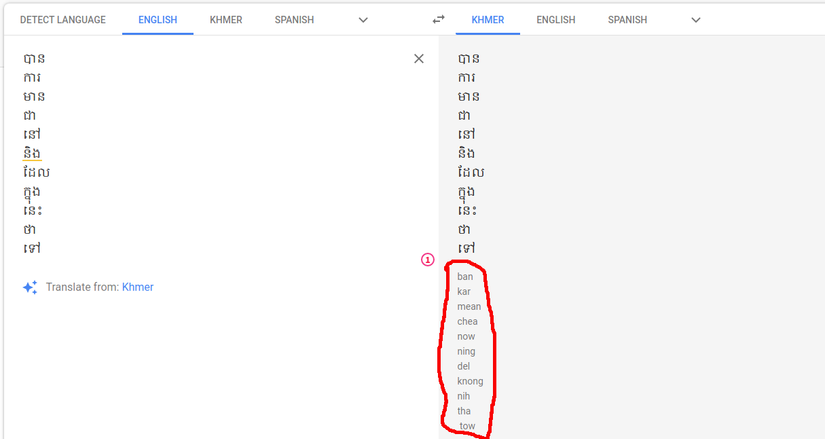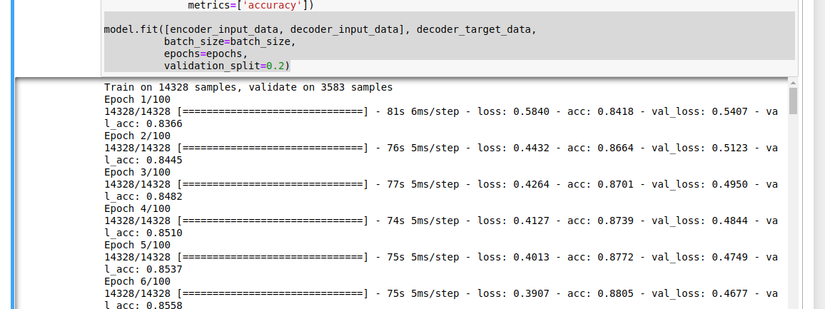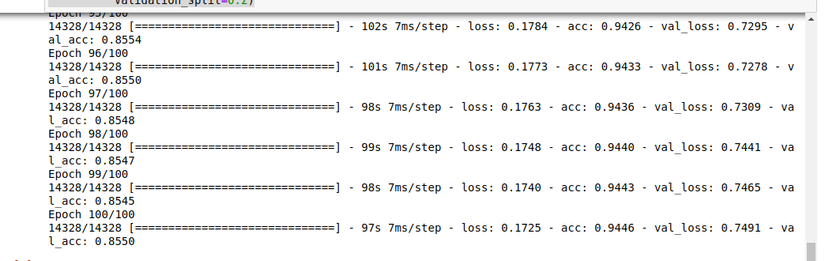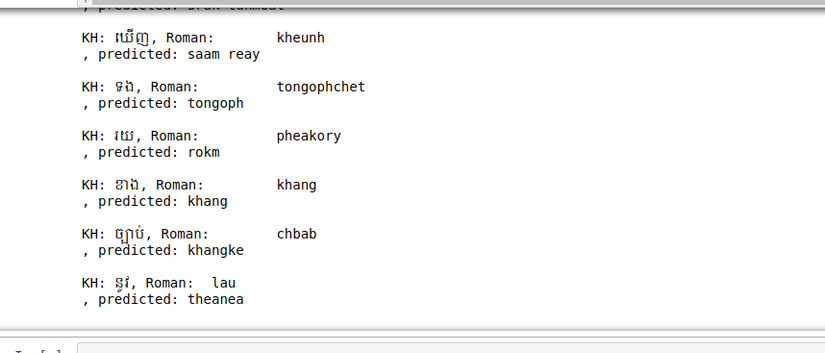# NLP: Khmer Romanization using Seq2Seq

### Introduction

In our previous article, we implemented and application to convert Khmer word into Roman by writing the logic from scratch following given paper since we didn't have enough data to apply deep learning for this problem. However, we notice that in googles translation, they also convert Khmer word into Roman. Therefore, we can easily use our Khmer words list in our previous article to get list of its Romanization. Then we can use these data to train our model for converting Khmer word to roman.### Plan of attack

There are many machines learning algorithms that we could use to solve our problem. Since, our problem is implementing a model to translate Khmer word to Roman, one particulate algorithm is very standout to this. It's Seq2Seq architecture. A Seq2Seq model is a model that takes a sequence of input (words, letters, time series, etc) and outputs another sequence of result. This model has achieved a lot of success in tasks like machine translation, text summarization, and image captioning. Google Translate started using such a model in production in late 2016. Moreover, we had also used this model for implementing our article about chatbot.### Implementation

For this experiment, we are using Keras for development our Seq2Seq model. Luckily, Keras also has a tutorial about build a model for translating English to French. We will modify those code to translate Khmer word to Roman instead. If there any lack of understand my code, you can go check the original code for more explaination here.

First, we import packages needed:

``````import numpy as np
import pandas as pd
from __future__ import print_function
from keras.models import Model
from keras.layers import Input, LSTM, Dense
``````

Then we load the data into memory using panda:

``````data_kh = pd.read_csv("data/data_kh.csv", header=None)
batch_size = 32  # Batch size for training.
epochs = 100  # Number of epochs to train for.
latent_dim = 256  # Latent dimensionality of the encoding space.
num_samples = 7154  # Number of samples to train on.
# Vectorize the data.
input_texts = []
target_texts = []
input_characters = set()
target_characters = set()
``````

Once data is loaded, we need to clear them and separeate it into unique individual character:

``````for input_text in data_kh:
input_text = str(input_text).strip()
input_texts.append(input_text)
for char in input_text:
if char not in input_characters:

for target_text in data_rom:
target_text = '\t' + str(target_text).strip() + '\n'
target_texts.append(target_text)
for char in str(target_text):
if char not in target_characters:

num_encoder_tokens = len(input_characters)
num_decoder_tokens = len(target_characters)
max_encoder_seq_length = max([len(txt) for txt in input_texts])
max_decoder_seq_length = max([len(txt) for txt in target_texts])
``````

Next, we init array for input and output sequences base on max length of input and output sample data.

``````input_token_index = dict(
[(char, i) for i, char in enumerate(input_characters)])
target_token_index = dict(
[(char, i) for i, char in enumerate(target_characters)])

encoder_input_data = np.zeros(
(len(input_texts), max_encoder_seq_length, num_encoder_tokens),
dtype='float32')
decoder_input_data = np.zeros(
(len(input_texts), max_decoder_seq_length, num_decoder_tokens),
dtype='float32')
decoder_target_data = np.zeros(
(len(input_texts), max_decoder_seq_length, num_decoder_tokens),
dtype='float32')
``````

Then, we encode/decode our input and out data before pass it into our model:

``````input_token_index = dict(
[(char, i) for i, char in enumerate(input_characters)])
target_token_index = dict(
[(char, i) for i, char in enumerate(target_characters)])

encoder_input_data = np.zeros(
(len(input_texts), max_encoder_seq_length, num_encoder_tokens),
dtype='float32')
decoder_input_data = np.zeros(
(len(input_texts), max_decoder_seq_length, num_decoder_tokens),
dtype='float32')
decoder_target_data = np.zeros(
(len(input_texts), max_decoder_seq_length, num_decoder_tokens),
dtype='float32')
``````

Using Keras we can build a seq2seq with ease:

``````# Define an input sequence and process it.
encoder_inputs = Input(shape=(None, num_encoder_tokens))
encoder = LSTM(latent_dim, return_state=True)
encoder_outputs, state_h, state_c = encoder(encoder_inputs)
# We discard `encoder_outputs` and only keep the states.
encoder_states = [state_h, state_c]

# Set up the decoder, using `encoder_states` as initial state.
decoder_inputs = Input(shape=(None, num_decoder_tokens))
# We set up our decoder to return full output sequences,
# and to return internal states as well. We don't use the
# return states in the training model, but we will use them in inference.
decoder_lstm = LSTM(latent_dim, return_sequences=True, return_state=True)
decoder_outputs, _, _ = decoder_lstm(decoder_inputs,
initial_state=encoder_states)
decoder_dense = Dense(num_decoder_tokens, activation='softmax')
decoder_outputs = decoder_dense(decoder_outputs)

# Define the model that will turn
# `encoder_input_data` & `decoder_input_data` into `decoder_target_data`
model = Model([encoder_inputs, decoder_inputs], decoder_outputs)

# Run training
model.compile(optimizer='rmsprop', loss='categorical_crossentropy',
metrics=['accuracy'])
``````

Then, we can start train our model:

``````
model.fit([encoder_input_data, decoder_input_data], decoder_target_data,
batch_size=batch_size,
epochs=epochs,
validation_split=0.2)
``````And don't forget to save our trained model if you don't to re-trin it again:

``````# Save model
# serialize model to JSON
model_json = model.to_json()
with open("model.json", "w") as json_file:
json_file.write(model_json)
model.save('s2s.h5')
``````

### Testing

Once training is complete, we now can test our model and check the result:

``````encoder_model = Model(encoder_inputs, encoder_states)

decoder_state_input_h = Input(shape=(latent_dim,))
decoder_state_input_c = Input(shape=(latent_dim,))
decoder_states_inputs = [decoder_state_input_h, decoder_state_input_c]
decoder_outputs, state_h, state_c = decoder_lstm(
decoder_inputs, initial_state=decoder_states_inputs)
decoder_states = [state_h, state_c]
decoder_outputs = decoder_dense(decoder_outputs)
decoder_model = Model(
[decoder_inputs] + decoder_states_inputs,
[decoder_outputs] + decoder_states)

reverse_input_char_index = dict(
(i, char) for char, i in input_token_index.items())
reverse_target_char_index = dict(
(i, char) for char, i in target_token_index.items())

def decode_sequence(input_seq):
# Encode the input as state vectors.
states_value = encoder_model.predict(input_seq)

# Generate empty target sequence of length 1.
target_seq = np.zeros((1, 1, num_decoder_tokens))
# Populate the first character of target sequence with the start character.
target_seq[0, 0, target_token_index['\t']] = 1.

# Sampling loop for a batch of sequences
# (to simplify, here we assume a batch of size 1).
stop_condition = False
decoded_sentence = ''
while not stop_condition:
output_tokens, h, c = decoder_model.predict(
[target_seq] + states_value)

# Sample a token
sampled_token_index = np.argmax(output_tokens[0, -1, :])
sampled_char = reverse_target_char_index[sampled_token_index]
decoded_sentence += sampled_char

# Exit condition: either hit max length
# or find stop character.
if (sampled_char == '\n' or
len(decoded_sentence) > max_decoder_seq_length):
stop_condition = True

# Update the target sequence (of length 1).
target_seq = np.zeros((1, 1, num_decoder_tokens))
target_seq[0, 0, sampled_token_index] = 1.

# Update states
states_value = [h, c]

return decoded_sentence

for seq_index in range(100):
# Take one sequence (part of the training set)
# for trying out decoding.
input_seq = encoder_input_data[seq_index: seq_index + 1]
decoded_sentence = decode_sequence(input_seq)
print('KH: ' + input_texts[seq_index] + ', Roman: ' + target_texts[seq_index] + ', predicted: ' + decoded_sentence)
``````

Let's run it.Base on the result, it seems our model is over fited. So, it your turn to improve this model to make it more awesome.

### What's next?

In article, we learned how to prepare our text data, and we create the model which will take the data we processed and use it to train translating Khmer word to Roman. We used an architecture called (seq2seq) or (Encoder Decoder), It is suitable for solving sequential problem. Where in our case the input sequence is Khmer words and our out put sequence is roman word where its length is different. However, our model is not produce good prediction yet and it's your turn to improve this model to compete with google.